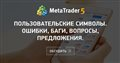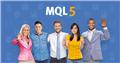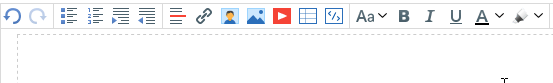# Any questions from newcomers on MQL4 and MQL5, help and discussion on algorithms and codes - page 981

jaffer wilson:Пользовательские символы. Ошибки, баги, вопросы, предложения.
• 2019.02.23
• www.mql5.com
Так как тема достаточна обширна, решил, что стоит выделить её в отдельное обсуждение...

Hello.

I am a newbie and I have the first problem - why does the compilation give an error? How to fix it?

//+------------------------------------------------------------------+
//|moy var1.mq4 |
#property version "1.00"
#property strict

extern int Magic = 111;
int CountSell()
//+------------------------------------------------------------------+
//| Expert initializationfunction|
//+-----------------------------------------------------------------+
int OnInit()
{
return(INIT_SUCCEEDED);
}
//+------------------------------------------------------------------+
//| Expert deinitialization function|
//+------------------------------------------------------------------+
void OnDeinit(const int reason)
{
}
//+------------------------------------------------------------------+
//| expert tick function|
//+------------------------------------------------------------------+
void OnTick()
{
{
int count =0;

for(int i = OrdersTotal() - 1; i >= 0; i--)
{
{
if(OrderSymbol() == Symbol() && OrderMagicNumber() == Magic && OrderType() == OP_SELL)
count++;
}
}
return;
}
}

//+------------------------------------------------------------------+

'int' - semicolon expected moy var1.mq4 13 1

1 error(s), 0 warning(s) 2 1Открой новые возможности в MetaTrader 5 с сообществом и сервисами MQL5
• www.mql5.com
Задавайте вопросы по техническому анализу, обсуждайте торговые системы и улучшайте свои навыки программирования торговых стратегий на языке MQL5. Общайтесь и обменивайтесь опытом на форуме с трейдерами всего мира и помогайте ответами новичкам — наше сообщество развивается вместе с вами. Хочу собрать ПК для работы с МТ 5, что посоветуете и...

petrovich1:

Hello.

I am a newbie and I have the first problem - why does the compilation give an error? How to fix it?

//+------------------------------------------------------------------+Afternoon. I cannot draw a Fibo object(OBJ_FIBO) in MT-4 without extending the lines to the right. When changing OBJPROP_RAY_RIGHT parameter, the ray is still drawn all the way to the right. And the tutorial script from the tutorial behaves the same way. I tried to change it to OBJPROP_RAY as for the segment, it doesn't work. If you are able to explain the reason. Thank you in advance.

Sergey Fionin:
Afternoon. I cannot draw a Fibo object ( OBJ_FIBO) in MT-4 without extending the lines to the right. When changing OBJPROP_RAY_RIGHT parameter, the ray is still drawn all the way to the right. And the tutorial script from the tutorial behaves in the same way. I tried to change it to OBJPROP_RAY as for the segment, it doesn't work. If you are able to explain the reason. Thank you in advance.

The matter is that MT4 is not MT5 and if the code compiles, it does not mean that it will work.

Alexey Viktorov :

The fact is that MT4 is not MT5, and if the code compiles, this does not mean that it will work.

I understand. And that's why I'm asking whether the setting " Continuation of the object to the right" by means of OBJPROP_RAY_RIGHT really works in MT-4 for ( OBJ_FIBO ) ? An example of a script is taken from a textbook for mt-4.

```#property strict
//--- описание
#property description "Скрипт строит графический объект \"Уровни Фибоначчи\"."
#property description "Координаты точек привязки задаются в процентах от"
#property description "размеров окна графика."
//--- покажем окно входных параметров при запуске скрипта
#property script_show_inputs
//--- входные параметры скрипта
input string           InpName= "FiboLevels" ;       // Имя объекта
input int              InpDate1= 10 ;               // Дата 1-ой точки в %
input int              InpPrice1= 65 ;               // Цена 1-ой точки в %
input int              InpDate2= 90 ;               // Дата 2-ой точки в %
input int              InpPrice2= 85 ;               // Цена 2-ой точки в %
input color            InpColor= clrRed ;           // Цвет объекта
input ENUM_LINE_STYLE InpStyle= STYLE_DASHDOTDOT ; // Стиль линии
input int              InpWidth= 1 ;                 // Толщина линии
input bool             InpBack= false ;             // Объект на заднем плане
input bool             InpSelection= true ;         // Выделить для перемещений
input bool             InpRayRight= false ;         // Продолжение объекта вправо
input bool             InpHidden= true ;             // Скрыт в списке объектов
input long             InpZOrder= 0 ;               // Приоритет на нажатие мышью
//+------------------------------------------------------------------+
//| Cоздает "Уровни Фибоначчи" по заданным координатам               |
//+------------------------------------------------------------------+
bool FiboLevelsCreate( const long             chart_ID= 0 ,         // ID графика
const string           name= "FiboLevels" , // имя объекта
const int              sub_window= 0 ,       // номер подокна
datetime               time1= 0 ,           // время первой точки
double                 price1= 0 ,           // цена первой точки
datetime               time2= 0 ,           // время второй точки
double                 price2= 0 ,           // цена второй точки
const color            clr= clrRed ,         // цвет объекта
const ENUM_LINE_STYLE style= STYLE_SOLID , // стиль линии объекта
const int              width= 1 ,           // толщина линии объекта
const bool             back= false ,         // на заднем плане
const bool             selection= true ,     // выделить для перемещений
const bool             ray_right= false ,   // продолжение объекта вправо
const bool             hidden= true ,       // скрыт в списке объектов
const long             z_order= 0 )         // приоритет на нажатие мышью
{
//--- установим координаты точек привязки, если они не заданы
ChangeFiboLevelsEmptyPoints(time1,price1,time2,price2);
//--- сбросим значение ошибки
ResetLastError ();
//--- создадим "Уровни Фибоначчи" по заданным координатам
if (! ObjectCreate (chart_ID,name, OBJ_FIBO ,sub_window,time1,price1,time2,price2))
{
Print ( __FUNCTION__ ,
": не удалось создать \"Уровни Фибоначчи\"! Код ошибки = " , GetLastError ());
return ( false );
}
//--- установим цвет
ObjectSetInteger (chart_ID,name, OBJPROP_COLOR ,clr);
//--- установим стиль линии
ObjectSetInteger (chart_ID,name, OBJPROP_STYLE ,style);
//--- установим толщину линии
ObjectSetInteger (chart_ID,name, OBJPROP_WIDTH ,width);
//--- отобразим на переднем (false) или заднем (true) плане
ObjectSetInteger (chart_ID,name, OBJPROP_BACK ,back);
//--- включим (true) или отключим (false) режим выделения объекта для перемещений
//--- при создании графического объекта функцией ObjectCreate, по умолчанию объект
//--- нельзя выделить и перемещать. Внутри же этого метода параметр selection
//--- по умолчанию равен true, что позволяет выделять и перемещать этот объект
ObjectSetInteger (chart_ID,name, OBJPROP_SELECTABLE ,selection);
ObjectSetInteger (chart_ID,name, OBJPROP_SELECTED ,selection);
//--- включим (true) или отключим (false) режим продолжения отображения объекта вправо
ObjectSetInteger (chart_ID,name, OBJPROP_RAY_RIGHT ,ray_right);
//--- скроем (true) или отобразим (false) имя графического объекта в списке объектов
ObjectSetInteger (chart_ID,name, OBJPROP_HIDDEN ,hidden);
//--- установи приоритет на получение события нажатия мыши на графике
ObjectSetInteger (chart_ID,name, OBJPROP_ZORDER ,z_order);
//--- успешное выполнение
return ( true );
}
//+------------------------------------------------------------------+
//| Задает количество уровней и их параметры                         |
//+------------------------------------------------------------------+
bool FiboLevelsSet( int              levels,             // количество линий уровня
double           &values[],         // значения линий уровня
color            &colors[],         // цвет линий уровня
ENUM_LINE_STYLE &styles[],         // стиль линий уровня
int              &widths[],         // толщина линий уровня
const long       chart_ID= 0 ,         // ID графика
const string     name= "FiboLevels" ) // имя объекта
{
//--- проверим размеры массивов
if (levels!= ArraySize (colors) || levels!= ArraySize (styles) ||
levels!= ArraySize (widths) || levels!= ArraySize (widths))
{
Print ( __FUNCTION__ , ": длина массива не соответствует количеству уровней, ошибка!" );
return ( false );
}
//--- установим количество уровней
ObjectSetInteger (chart_ID,name, OBJPROP_LEVELS ,levels);
//--- установим свойства уровней в цикле
for ( int i= 0 ;i<levels;i++)
{
//--- значение уровня
ObjectSetDouble (chart_ID,name, OBJPROP_LEVELVALUE ,i,values[i]);
//--- цвет уровня
ObjectSetInteger (chart_ID,name, OBJPROP_LEVELCOLOR ,i,colors[i]);
//--- стиль уровня
ObjectSetInteger (chart_ID,name, OBJPROP_LEVELSTYLE ,i,styles[i]);
//--- толщина уровня
ObjectSetInteger (chart_ID,name, OBJPROP_LEVELWIDTH ,i,widths[i]);
//--- описание уровня
ObjectSetString (chart_ID,name, OBJPROP_LEVELTEXT ,i, DoubleToString ( 100 *values[i], 1 ));
}
//--- успешное выполнение
return ( true );
}
//+------------------------------------------------------------------+
//| Перемещает точку привязки "Уровней Фибоначчи"                    |
//+------------------------------------------------------------------+
bool FiboLevelsPointChange( const long    chart_ID= 0 ,         // ID графика
const string name= "FiboLevels" , // имя объекта
const int     point_index= 0 ,     // номер точки привязки
datetime      time= 0 ,             // координата времени точки привязки
double        price= 0 )           // координата цены точки привязки
{
//--- если координаты точки не заданы, то перемещаем ее на текущий бар с ценой Bid
if (!time)
time= TimeCurrent ();
if (!price)
price= SymbolInfoDouble ( Symbol (), SYMBOL_BID );
//--- сбросим значение ошибки
ResetLastError ();
//--- переместим точку привязки
if (! ObjectMove (chart_ID,name,point_index,time,price))
{
Print ( __FUNCTION__ ,
": не удалось переместить точку привязки! Код ошибки = " , GetLastError ());
return ( false );
}
//--- успешное выполнение
return ( true );
}
//+------------------------------------------------------------------+
//| Удаляет "Уровни Фибоначчи"                                       |
//+------------------------------------------------------------------+
bool FiboLevelsDelete( const long    chart_ID= 0 ,         // ID графика
const string name= "FiboLevels" ) // имя объекта
{
//--- сбросим значение ошибки
ResetLastError ();
//--- удалим объект
if (! ObjectDelete (chart_ID,name))
{
Print ( __FUNCTION__ ,
": не удалось удалить \"Уровни Фибоначчи\"! Код ошибки = " , GetLastError ());
return ( false );
}
//--- успешное выполнение
return ( true );
}
//+------------------------------------------------------------------+
//| Проверяет значения точек привязки "Уровней Фибоначчи" и для      |
//| пустых значений устанавливает значения по умолчанию              |
//+------------------------------------------------------------------+
void ChangeFiboLevelsEmptyPoints( datetime &time1, double &price1,
datetime &time2, double &price2)
{
//--- если время второй точки не задано, то она будет на текущем баре
if (!time2)
time2= TimeCurrent ();
//--- если цена второй точки не задана, то она будет иметь значение Bid
if (!price2)
price2= SymbolInfoDouble ( Symbol (), SYMBOL_BID );
//--- если время первой точки не задано, то она лежит на 9 баров левее второй
if (!time1)
{
//--- массив для приема времени открытия 10 последних баров
datetime temp[ 10 ];
CopyTime ( Symbol (), Period (),time2, 10 ,temp);
//--- установим первую точку на 9 баров левее второй
time1=temp[ 0 ];
}
//--- если цена первой точки не задана, то сдвинем ее на 200 пунктов ниже второй
if (!price1)
price1=price2- 200 * SymbolInfoDouble ( Symbol (), SYMBOL_POINT );
}
//+------------------------------------------------------------------+
//| Script program start function                                    |
//+------------------------------------------------------------------+
void OnStart ()
{
//--- проверим входные параметры на корректность
if (InpDate1< 0 || InpDate1> 100 || InpPrice1< 0 || InpPrice1> 100 ||
InpDate2< 0 || InpDate2> 100 || InpPrice2< 0 || InpPrice2> 100 )
{
Print ( "Ошибка! Некорректные значения входных параметров!" );
return ;
}
//--- количество видимых баров в окне графика
int bars=( int ) ChartGetInteger ( 0 , CHART_VISIBLE_BARS );
//--- размер массива price
int accuracy= 1000 ;
//--- массивы для хранения значений дат и цен, которые будут использованы
//--- для установки и изменения координат точек привязки "Уровней Фибоначчи"
datetime date[];
double    price[];
//--- выделение памяти
ArrayResize (date,bars);
ArrayResize (price,accuracy);
//--- заполним массив дат
ResetLastError ();
if ( CopyTime ( Symbol (), Period (), 0 ,bars,date)==- 1 )
{
Print ( "Не удалось скопировать значения времени! Код ошибки = " , GetLastError ());
return ;
}
//--- заполним массив цен
//--- найдем максимальное и минимальное значение графика
double max_price= ChartGetDouble ( 0 , CHART_PRICE_MAX );
double min_price= ChartGetDouble ( 0 , CHART_PRICE_MIN );
//--- определим шаг изменения цены и заполним массив
double step=(max_price-min_price)/accuracy;
for ( int i= 0 ;i<accuracy;i++)
price[i]=min_price+i*step;
//--- определим точки для рисования "Уровней Фибоначчи"
int d1=InpDate1*(bars- 1 )/ 100 ;
int d2=InpDate2*(bars- 1 )/ 100 ;
int p1=InpPrice1*(accuracy- 1 )/ 100 ;
int p2=InpPrice2*(accuracy- 1 )/ 100 ;
//--- создадим объект
if (!FiboLevelsCreate( 0 ,InpName, 0 ,date[d1],price[p1],date[d2],price[p2],InpColor,
InpStyle,InpWidth,InpBack,InpSelection,InpRayRight,InpHidden,InpZOrder))
{
return ;
}
//--- перерисуем график и подождем 1 секунду
ChartRedraw ();
Sleep ( 1000 );
//--- теперь будем перемещать точки привязки
//--- счетчик цикла
int v_steps=accuracy* 2 / 5 ;
//--- перемещаем первую точку привязки
for ( int i= 0 ;i<v_steps;i++)
{
//--- возьмем следующее значение
if (p1> 1 )
p1-= 1 ;
//--- сдвигаем точку
if (!FiboLevelsPointChange( 0 ,InpName, 0 ,date[d1],price[p1]))
return ;
//--- проверим факт принудительного завершения скрипта
if ( IsStopped ())
return ;
//--- перерисуем график
ChartRedraw ();
}
//--- задержка в 1 секунду
Sleep ( 1000 );
//--- счетчик цикла
v_steps=accuracy* 4 / 5 ;
//--- перемещаем вторую точку привязки
for ( int i= 0 ;i<v_steps;i++)
{
//--- возьмем следующее значение
if (p2> 1 )
p2-= 1 ;
//--- сдвигаем точку
if (!FiboLevelsPointChange( 0 ,InpName, 1 ,date[d2],price[p2]))
return ;
//--- проверим факт принудительного завершения скрипта
if ( IsStopped ())
return ;
//--- перерисуем график
ChartRedraw ();
}
//--- задержка в 1 секунду
Sleep ( 1000 );
//--- удалим объект с графика
FiboLevelsDelete( 0 ,InpName);
ChartRedraw ();
//--- задержка в 1 секунду
Sleep ( 1000 );
//---
}

```

Sergey Fionin:

I understand. That's why I'm asking if setting " Continue object to the right" with OBJPROP_RAY_RIGHT really works in mt-4 for ( OBJ_FIBO)? The example scriptis taken from the tutorial for mt-4.

It is possible that they missed it when editing the help. It was corrected from mql5 documentation.

Hi all. It's been a week since I started studying mql and now I'm puzzling over how to implement such a mechanism. The chart has a button(OBJ_BUTTON), how and what should be written in the code, so that when you click the button would be derived from a given sequence of numbers in order. I.e., there is a sequence of 2, 4, 8, 16, 32, 64, 128. I, for example, press the button on the chart and the number from this sequence should be displayed in a comment, strictly in order. I pressed the button - it will show 2, I pressed it again - it will show 4 and so on. And by pressing the second button, for example, these numbers would appear in reverse order. If you can write a working code, my joy will be over)))

Ivan Revedzhuk:
Hi all. It's been a week since I started studying mql, and I've been puzzling over how to implement such a mechanism. The chart has a button ( OBJ_BUTTON), how and what should be written in the code to print a number from a given sequence in order when you click on the button. I.e., there is a sequence of 2, 4, 8, 16, 32, 64, 128. I, for example, press the button on the chart and the number from this sequence should be displayed in a comment, strictly in order. I pressed the button - it will show 2, I pressed it again - it will show 4 and so on. And by pressing the second button, for example, these numbers would appear in reverse order. If you can write a working code, my joy will be over)))
```if(ObjectGetInteger(0, "name", OBJPROP_STATE) == true)
// Кнопка нажата
// Вывести в Comment какое нужно число

// Отжать кнопку
ObjectSetInteger(0, "name", OBJPROP_STATE) == false

```

To determine what number is in the comment, read the graph documentation. There is a function there to read the comment.

Or the easiest, this number to put in a static variable, and it in Comment()

Alexey Viktorov:

To determine what number is in the comment, read the graph documentation. There is a function there to read the comment.

Or the easiest way is to stick this number in a static variable and put it in Comment()

Thanks for the reply. I've found how to do button press handling in the help, but I'm having trouble with the rest. I'm working not just with one number, but with a sequence of integers and I need one push of the button to output a number from this sequence, in order from 2 to 128. The question is exactly how to do this...